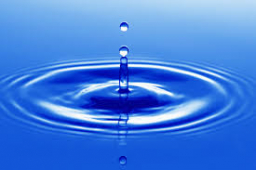# Temperature 61484

The air bubble at the bottom of the lake at a depth of h = 21 m has a radius r1 = 1 cm at a temperature of t1 = 4 °C. The bubble rises slowly to the surface, and its volume increases. Calculate its radius when it reaches the lake's surface, with a temperature of t2 = 27 °C. Atmospheric pressure is b = 0.1 MPa, water density = 1030 kg. m-3. Do not take surface tension into account.

r2 =  1.0269 cm

### Step-by-step explanation:Did you find an error or inaccuracy? Feel free to write us. Thank you!

Tips for related online calculators
Need help calculating sum, simplifying, or multiplying fractions? Try our fraction calculator.
Do you know the volume and unit volume, and want to convert volume units?
Do you want to convert mass units?

#### Grade of the word problem:

We encourage you to watch this tutorial video on this math problem: